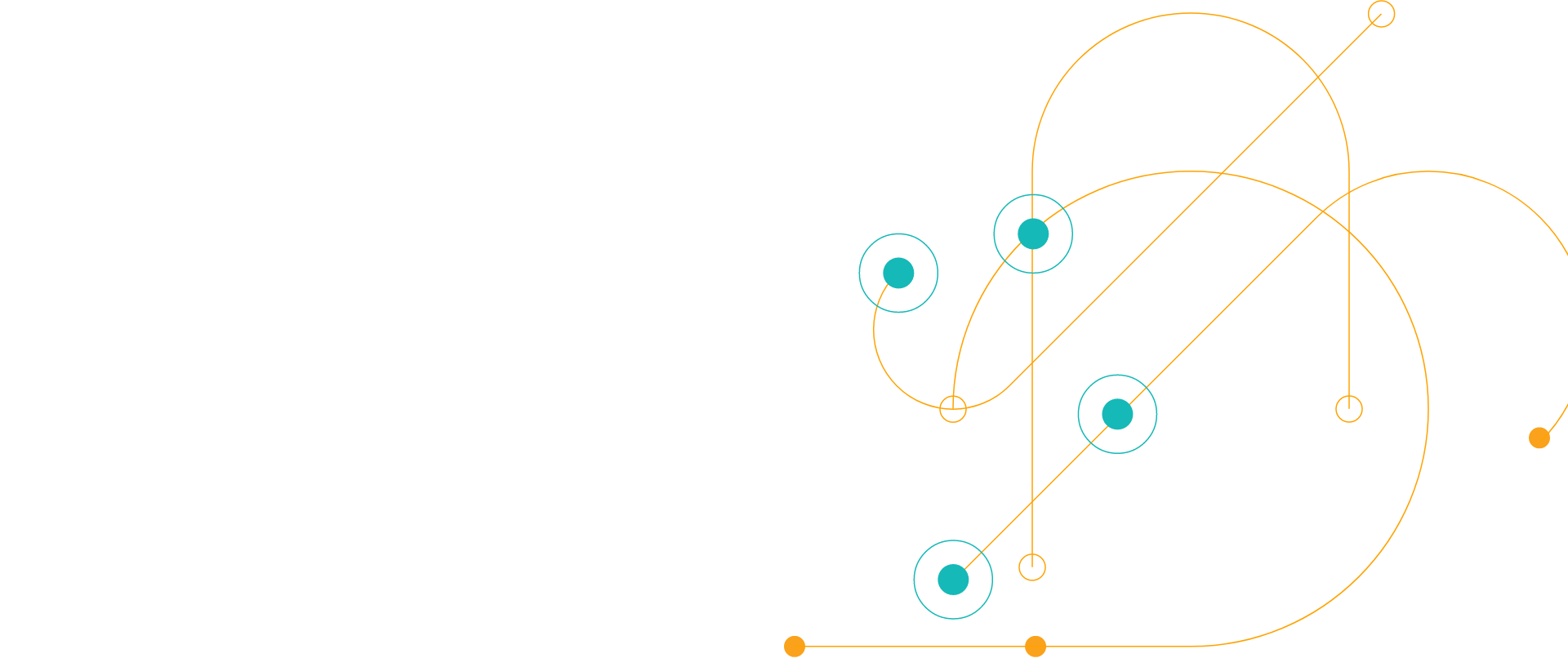# Chef Desktop for the Distributed Workforce

Back to eBooks

### Empower your organization with Chef Desktop

As Work from Home (WFH) is becoming the new reality of work for most of the world, the management of the laptops has become a problem for most organizations.

Business can now adopt a single, automated solution to manage their IT resources, that allows them to:

• Apply consistent, flexible policy-driven configuration to their fleet
• Eliminate time-consuming manual processes and reduce costs
• Maintain visibility into fleet-wide status and compliance state independent of OS (macOS, Windows, Linux)
• Audit and remediate against industry standards (CIS/DISA-STIGs)

<div class='-tac -pb2'> <div class='svgi -c-magenta -mb1'> <svg xmlns='http://www.w3.org/2000/svg' width='178.099' height='129.473' viewBox='0 0 178.099 129.473'><defs><style>.a{fill:none;stroke:#d91683;stroke-miterlimit:10;stroke-width:2px;}</style></defs><g transform='translate(-964 -388)'><g transform='translate(2053.531 592.899)'><path class='a' d='M-1001.416-112.527h0a15.244,15.244,0,0,0,15.242-15.244v-7.543a2.33,2.33,0,0,1,2.332-2.33h1.15a7.628,7.628,0,0,1,7.628,7.627v17.49h12.3a7.469,7.469,0,0,1,7.469,7.469,7.481,7.481,0,0,1-.291,2.065l-5.254,18.267a7.47,7.47,0,0,1-7.178,5.4h-23.82'/><path class='a' d='M-1016.167-89.561v-22.586a5.337,5.337,0,0,1,5.337-5.339h4.075a5.339,5.339,0,0,1,5.339,5.339v36.721'/><circle class='a' cx='2.418' cy='2.418' r='2.418' transform='translate(-1027.341 -153.295)'/><circle class='a' cx='3.089' cy='3.089' r='3.089' transform='translate(-932.889 -108.485)'/><circle class='a' cx='3.089' cy='3.089' r='3.089' transform='translate(-1045.892 -148.25)'/><line class='a' transform='translate(-1032.363 -112.148)'/><line class='a' x1='7.549' transform='translate(-1024.031 -112.148)'/><line class='a' x1='11.31' transform='translate(-1027.341 -79.57)'/><line class='a' y1='30.648' transform='translate(-982.378 -199.62)'/><line class='a' y1='9.209' transform='translate(-987.339 -204.899)'/><line class='a' x2='14.429' transform='translate(-945.128 -133.443)'/><line class='a' x2='16.313' transform='translate(-1029.224 -133.443)'/><line class='a' x1='17.459' y1='17.459' transform='translate(-1024.252 -173.651)'/><line class='a' y1='17.459' x2='17.459' transform='translate(-954.858 -173.657)'/><line class='a' x2='15.442' transform='translate(-926.874 -133.443)'/><circle class='a' cx='5.045' cy='5.045' r='5.045' transform='translate(-924.198 -158.341)'/><circle class='a' cx='3.93' cy='3.93' r='3.93' transform='translate(-956.811 -203.551)'/><path class='a' d='M-1002.58-130.114a21.3,21.3,0,0,1,21.3-21.295,21.3,21.3,0,0,1,21.295,21.295'/><path class='a' d='M-1007.275-130.674a25.99,25.99,0,0,1,25.99-25.99,25.99,25.99,0,0,1,25.99,25.99'/><path class='a' d='M-1022.911-112.861a32.811,32.811,0,0,1-32.81,32.81,32.811,32.811,0,0,1-32.81-32.81'/><circle class='a' cx='25.308' cy='25.308' r='25.308' transform='translate(-1081.029 -137.456)'/><path class='a' d='M-1065.55-107.007l9.829,7.8,9.829-7.8'/><line class='a' y2='25.892' transform='translate(-1055.721 -125.094)'/><circle class='a' cx='0.565' cy='0.565' r='0.565' transform='translate(-969.36 -164.564)'/><circle class='a' cx='0.565' cy='0.565' r='0.565' transform='translate(-945.692 -110.858)'/><line class='a' x1='3.557' y2='3.557' transform='translate(-939.276 -184.213)'/><line class='a' x1='9.946' y2='9.946' transform='translate(-934.773 -186.311)'/><line class='a' x1='9.946' y2='9.946' transform='translate(-937.266 -173.945)'/><line class='a' x1='9.946' y1='9.946' transform='translate(-1039.929 -186.311)'/><line class='a' x1='9.946' y1='9.946' transform='translate(-1037.436 -173.945)'/><circle class='a' cx='2.605' cy='2.605' r='2.605' transform='translate(-1012.516 -82.175)'/></g></g></svg> </div> <h3>Thank You</h3> <p>If your download hasn't started in 5 seconds please</p> <p><a data-sf-ec-immutable='' href='https://d1l5pp53ux74mz.cloudfront.net/docs/default-source/ebook/chef_desktop_ebook_distributed_work_environments_are_the_new_reality.pdf?sfvrsn=6d17ff07_2' class='-i-next' target='_blank' rel='nofollow'>Click here to download </a></p> </div> <script>document.getElementsByClassName('js-form-title').style.display='none';var win=window.open('https://d1l5pp53ux74mz.cloudfront.net/docs/default-source/ebook/chef_desktop_ebook_distributed_work_environments_are_the_new_reality.pdf?sfvrsn=6d17ff07_2', '_blank');win.focus();</script><script>if (typeof (window.dataLayer) !== 'undefined') { window.dataLayer = window.dataLayer || []; dataLayer.push({'event': 'custom.successMessageShown'}); } </script>